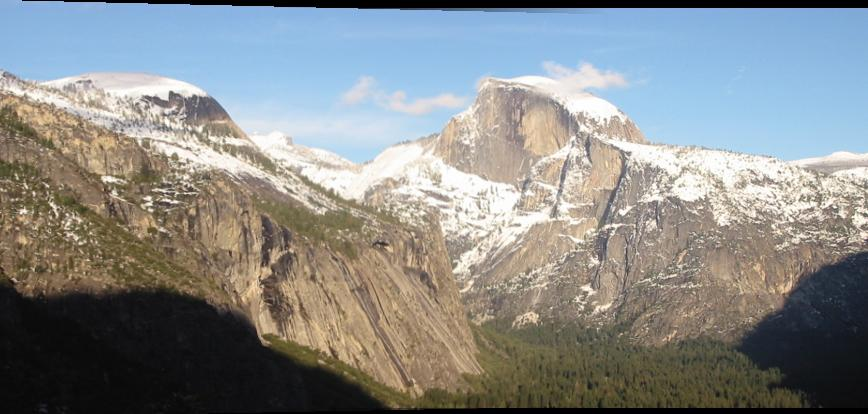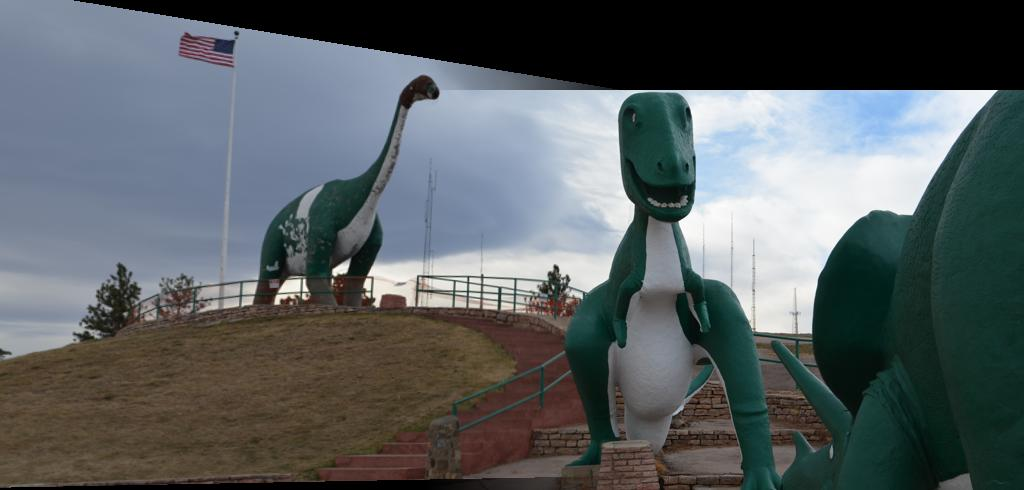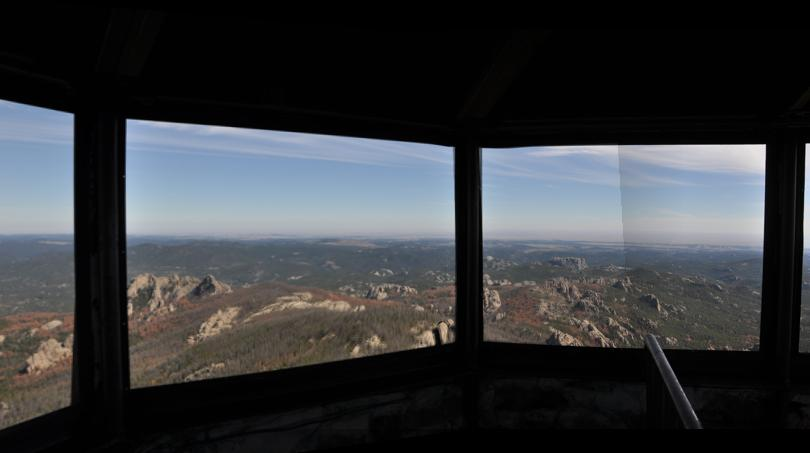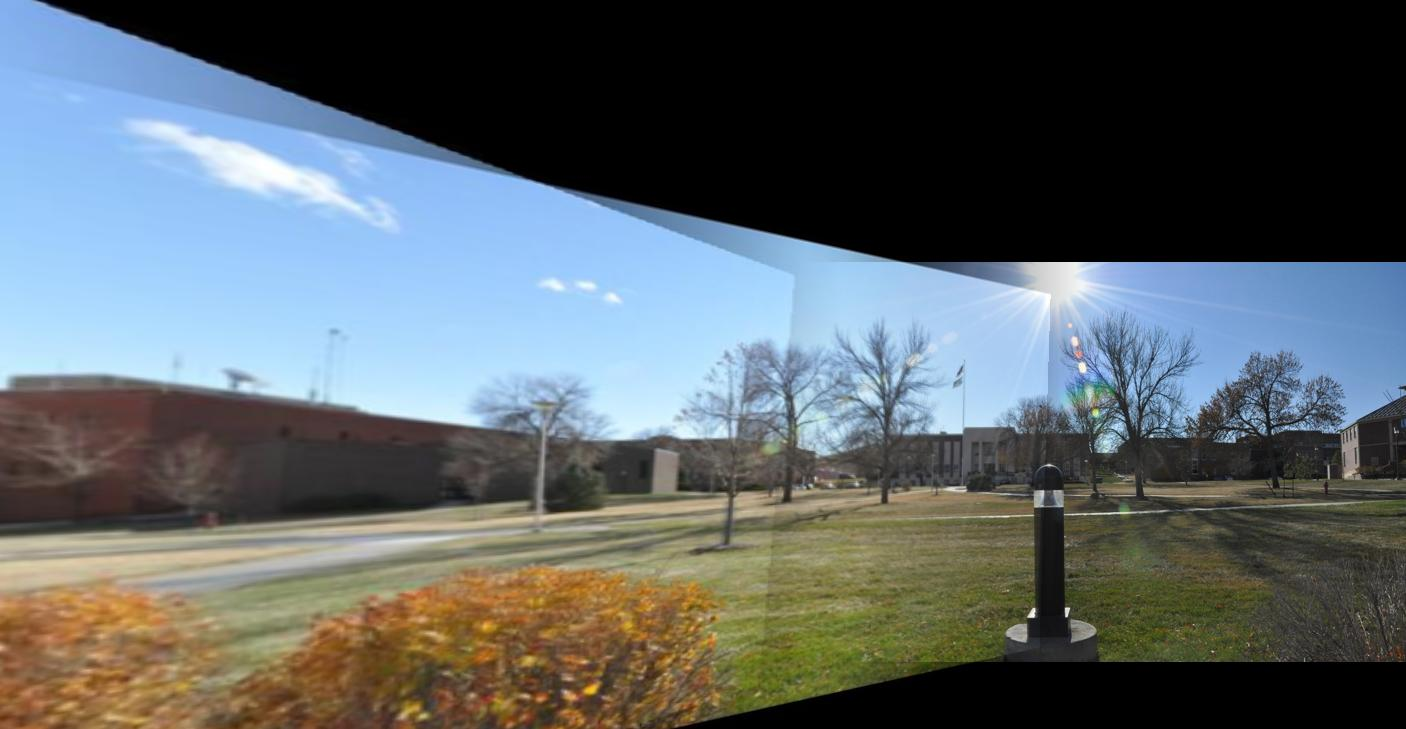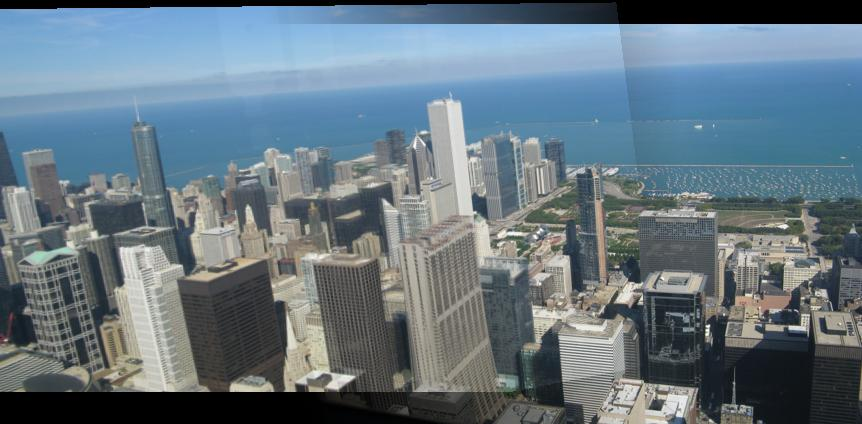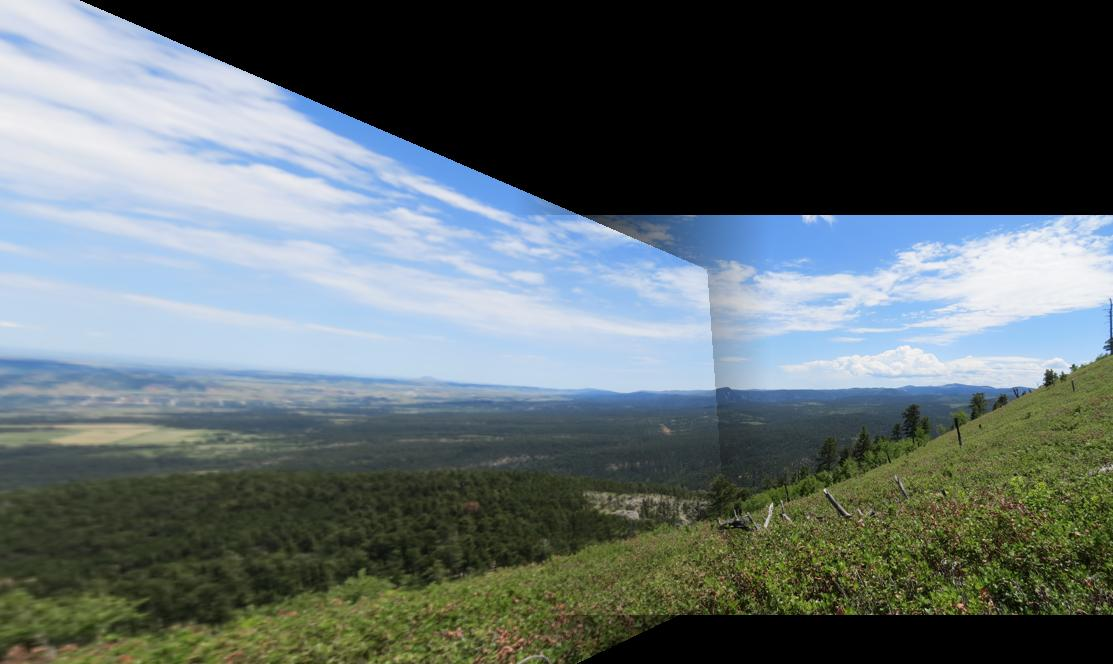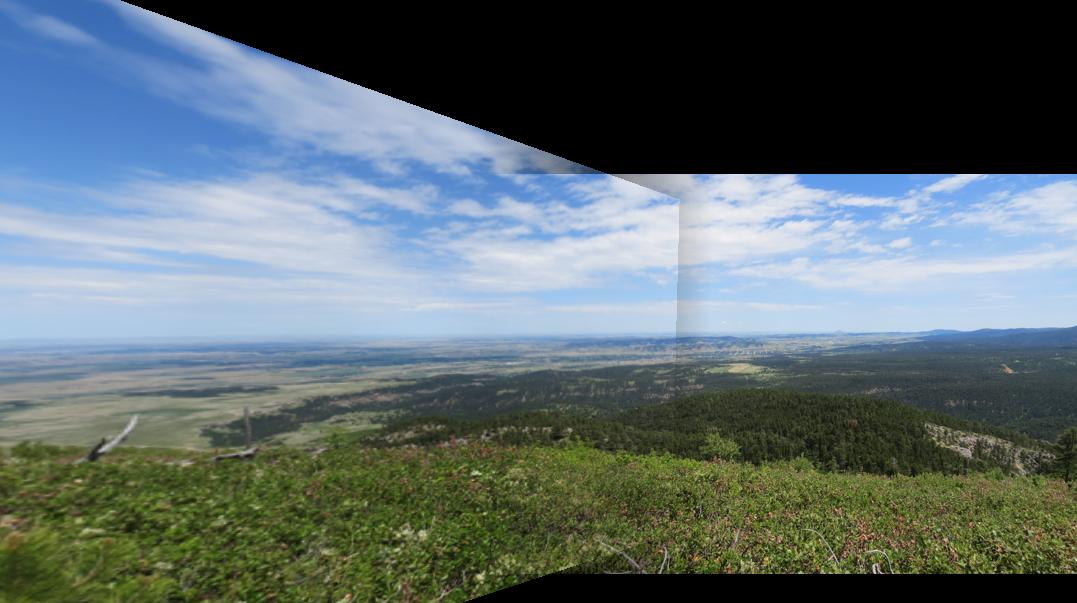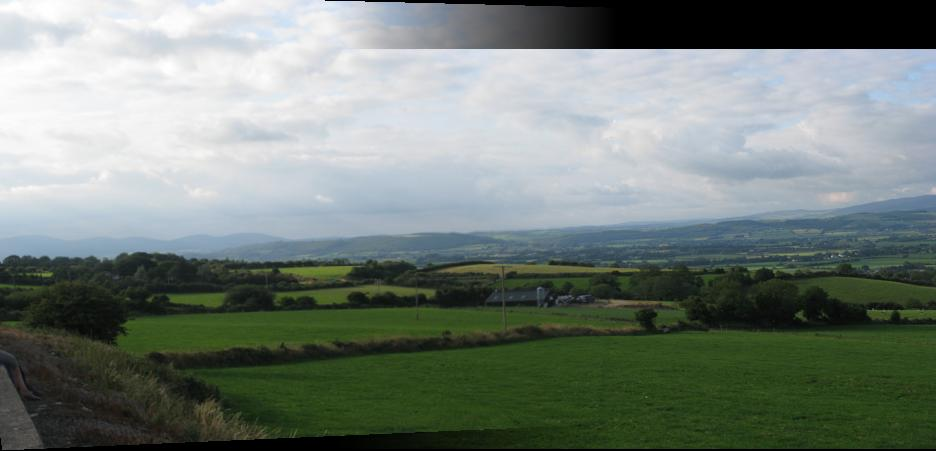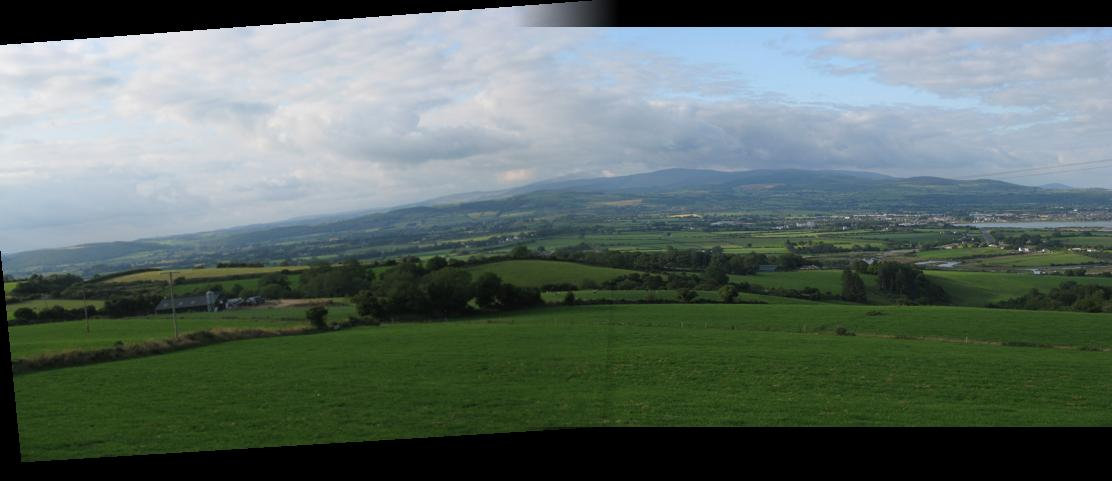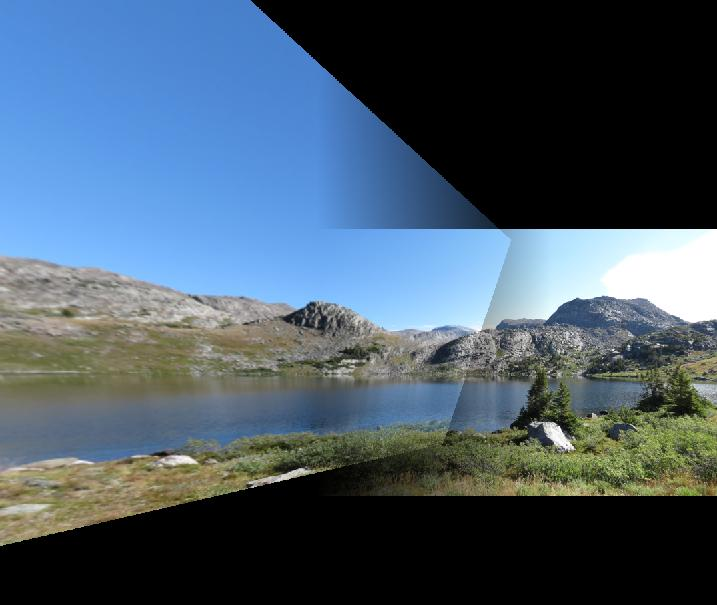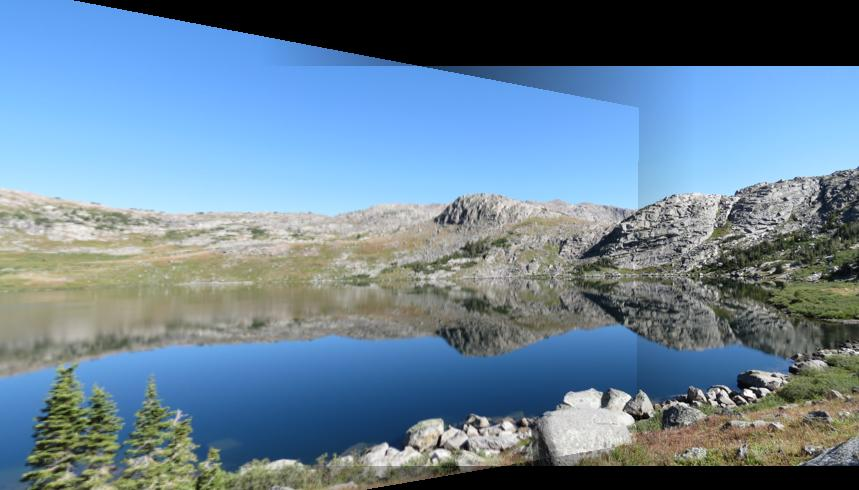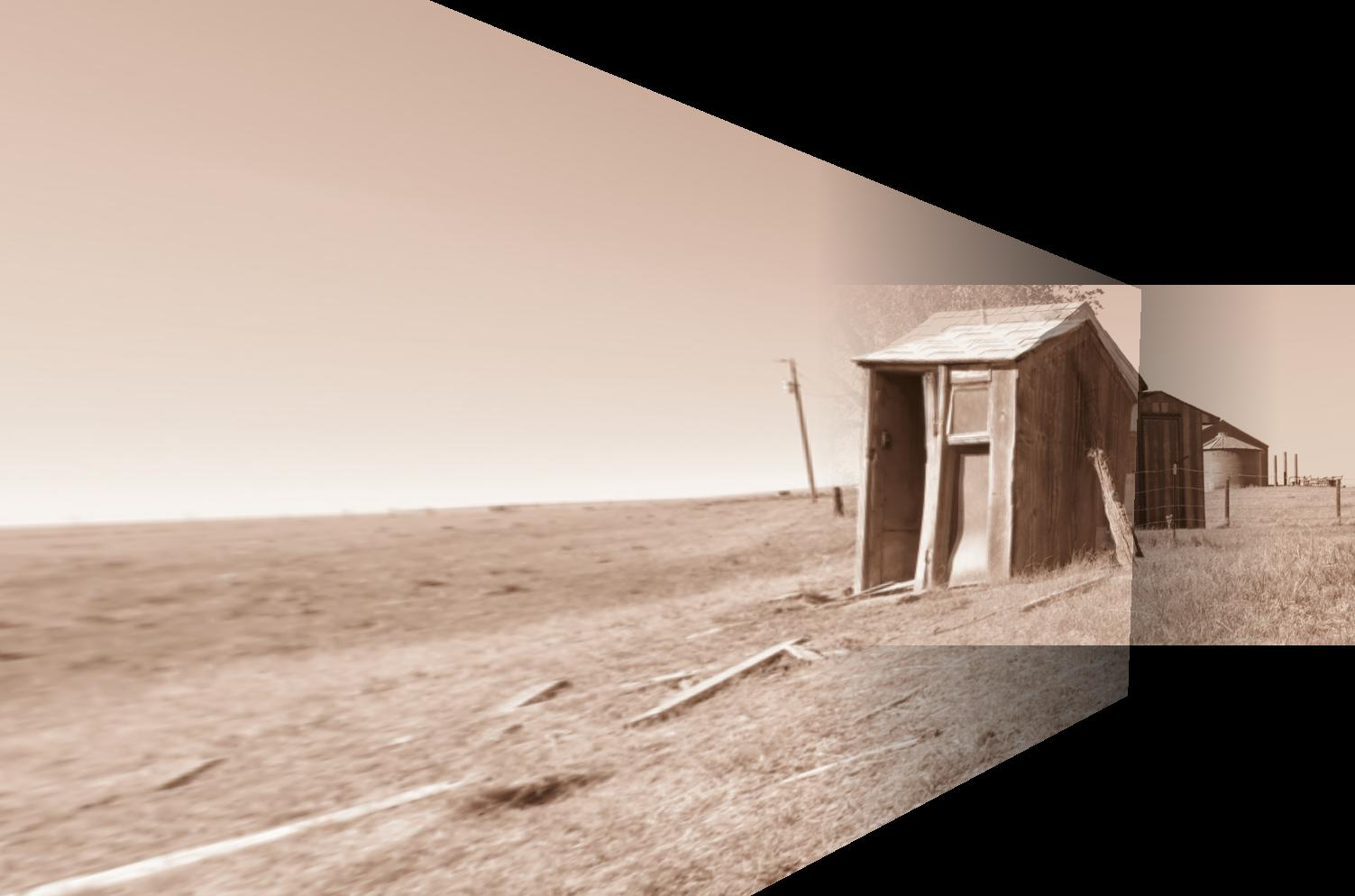Most cameras and cell phones today have the ability to combine multiple images or create on panoramic view. To be able to stitch images together, they must be transformed into the same frame. This is done by finding the same points in each image and creating a homography matrix to relate the points from one image to the other. Once the homography matrix is found, it can be used to morph one image into the same frame as the other and then combine the images.

## Getting Matches

There are two methods for finding matching points.

### Manual Selection

I originally added this feature to the project for testing purposes. I left it included because it created extra functionality. Manually selecting the points can be used to overlay one image onto another. It could also be used to select the points for stitching the images together but it’s very difficult to get the correct matching pixels in each image.

### SIFT

The SIFT function was added using a program created by David Lowe which is very accurate at finding matches. The originally functionally would show two images with lines drawn between the matching images. It was modified to return the matches to be used for calculating the homogenous matrix.

## Computing the Homography Matrix

The homography matrix represents a function to transform point in one image’s frame to another’s.

 ``````1 `````` ``````p’ = Hp ``````

Where p is a matrix of the original points, H is the homography matrix, and p’ is the resulting transformed image.

### Singular Value Decomposition (SVD)

The homography matrix is calculated using a minimum of four points. Using more points can lead to a greater number of matches but less perfect matches. SVD puts a matrix into the form:

 ``````1 `````` ``````A=UEV^T ``````

The right most column of V^T is our homography matrix that will need to be shaped into the standard form.

### Checking for Accuracy

The quality of the homography matrix is found using the RANSAC algorithm. The homography matrix is first calculated four randomly selected matching points. Every point in P is then transformed to P` using the homography matrix. The resulting points are then compared to the original points from the match. If the difference is within a threshold then the match is recorded in a separate list. If enough matches are added to the list, then the homography matrix is recalculated using the points in this list and the process is restarted. The process repeats multiple times and the best homography matrix is saved.

## Warping

With the homography matrix calculated, the two images can now be combined. The corners of the image to be warped are found first so that we know the dimensions of the final warped image. A mesh grid is created using the dimensions of the warped corners. The inverse homography matrix is applied to these in order to eliminate holes and se what points from the original matrix can be warped. Finally, interpolation is used to copy points from the original image into the warped frame.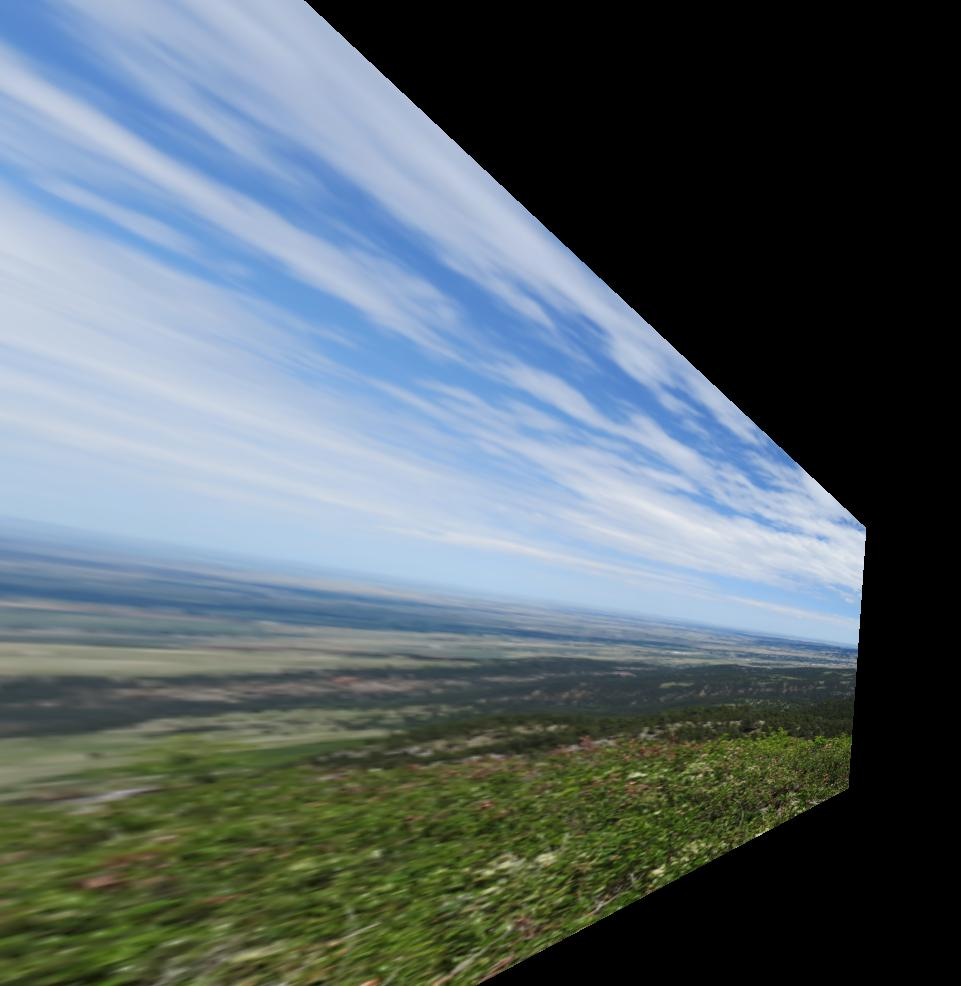## Stitching

We now have an original image and the other image to be warped into that image’s frame. The original image is placed into a matrix first. The warped image then overrides any similar points as it is appended to the matrix. This results in a crisp edge where the two images meet.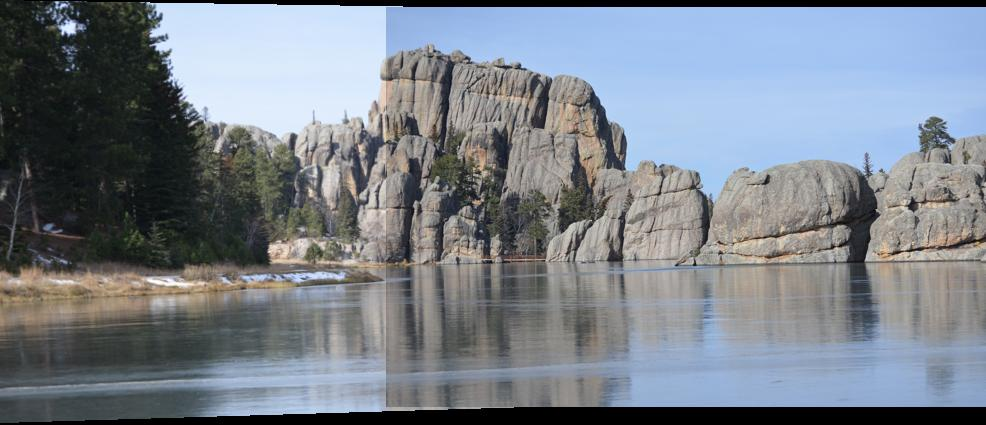### Feathering

Feathering solves the crisp edge that is created during the stitching step. This is applied anywhere that the two overlayed images overlapped. This works by causing the images to “fade out” as they move further from their center. The overlapping sections are multiplied by an evenly spaced vector going from 0 to 1 or 1 to 0 depending on the direction of the image. The pixels closer to 1 keep most of their magnitude while the pixels closer to 0 lose most of their magnitude.## Results

The resulting images can be seen below. Most of these are the benchmark images that were required to be used but I also used a number of my own personal images. These images had very good results andI think they are very interesting to look at. The most interesting are the images that were taken at different angles but the program still managed to stitch them together.